# Fundamental Trigonometric Identities How do we use fundamental

• Slides: 9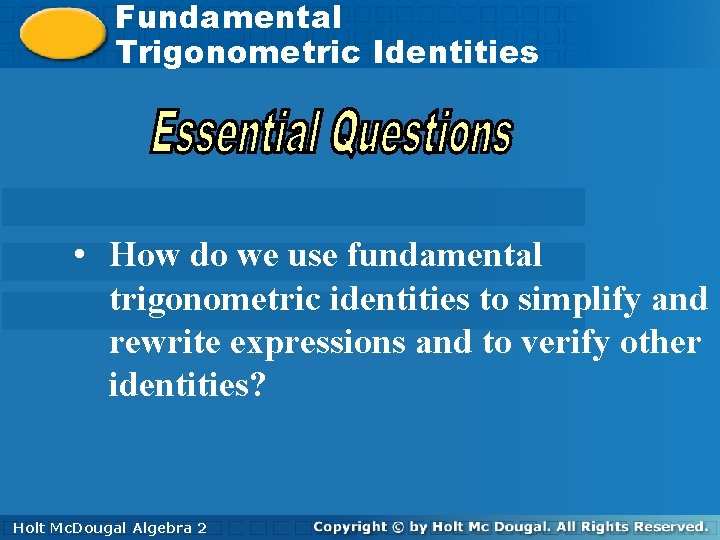Fundamental. Trigonometric Identities • How do we use fundamental trigonometric identities to simplify and rewrite expressions and to verify other identities? Holt. Mc. Dougal Algebra 2 HoltFundamental Trigonometric Identities You can use the fundamental trigonometric identities to simplify expressions. Helpful Hint If you get stuck, try converting all of the trigonometric functions to sine and cosine functions. Holt Mc. Dougal Algebra 2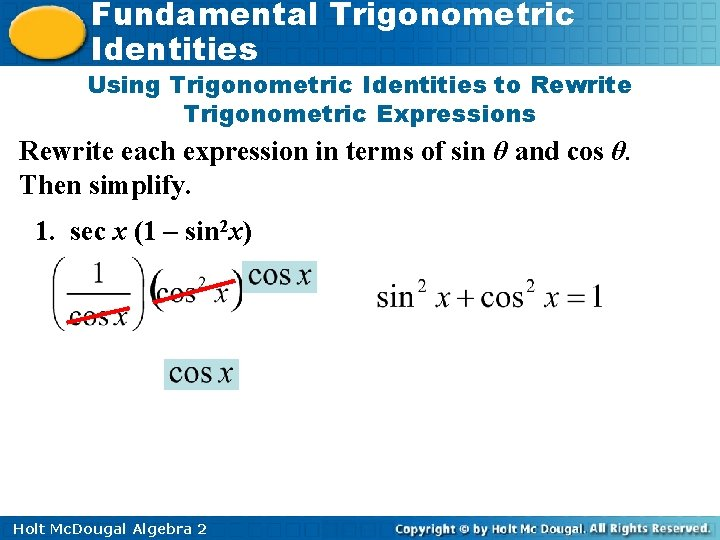Fundamental Trigonometric Identities Using Trigonometric Identities to Rewrite Trigonometric Expressions Rewrite each expression in terms of sin θ and cos θ. Then simplify. 1. sec x (1 – sin 2 x) Holt Mc. Dougal Algebra 2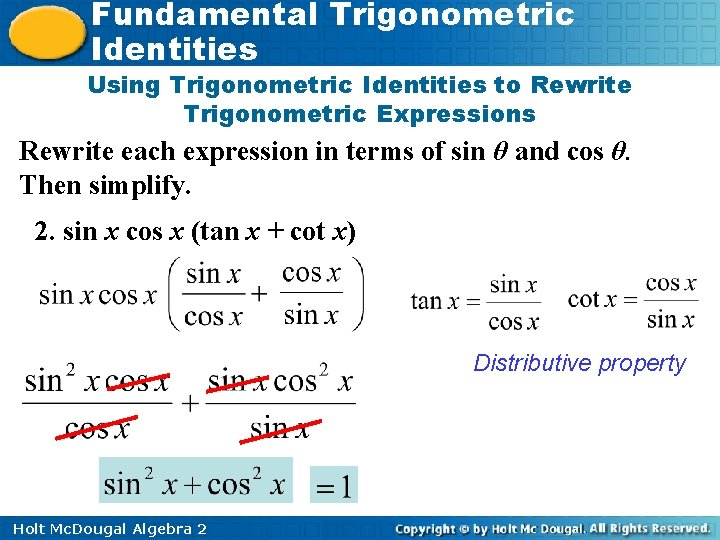Fundamental Trigonometric Identities Using Trigonometric Identities to Rewrite Trigonometric Expressions Rewrite each expression in terms of sin θ and cos θ. Then simplify. 2. sin x cos x (tan x + cot x) Distributive property Holt Mc. Dougal Algebra 2Fundamental Trigonometric Identities Using Trigonometric Identities to Rewrite Trigonometric Expressions Rewrite each expression in terms of sin θ and cos θ. Then simplify. Factor the numerator Holt Mc. Dougal Algebra 2Fundamental Trigonometric Identities Using Trigonometric Identities to Rewrite Trigonometric Expressions Rewrite each expression in terms of sin θ and cos θ. Then simplify. Holt Mc. Dougal Algebra 2Fundamental Trigonometric Identities Using Trigonometric Identities to Rewrite Trigonometric Expressions Rewrite each expression in terms of sin θ and cos θ. Then simplify. Holt Mc. Dougal Algebra 2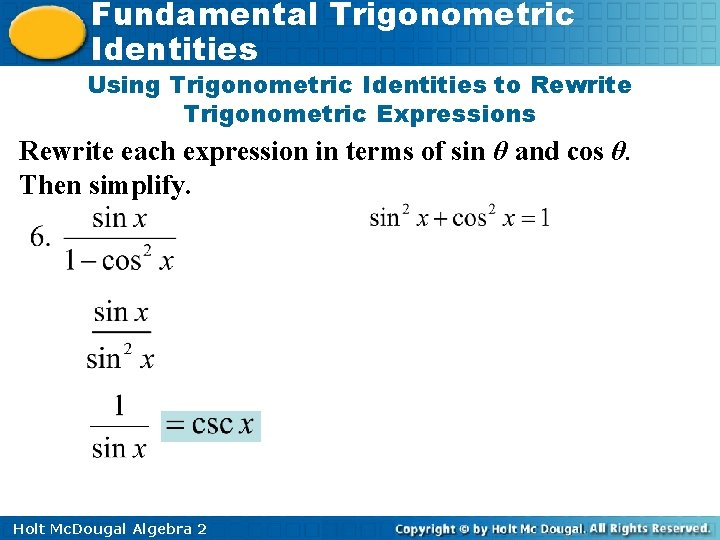Fundamental Trigonometric Identities Using Trigonometric Identities to Rewrite Trigonometric Expressions Rewrite each expression in terms of sin θ and cos θ. Then simplify. Holt Mc. Dougal Algebra 2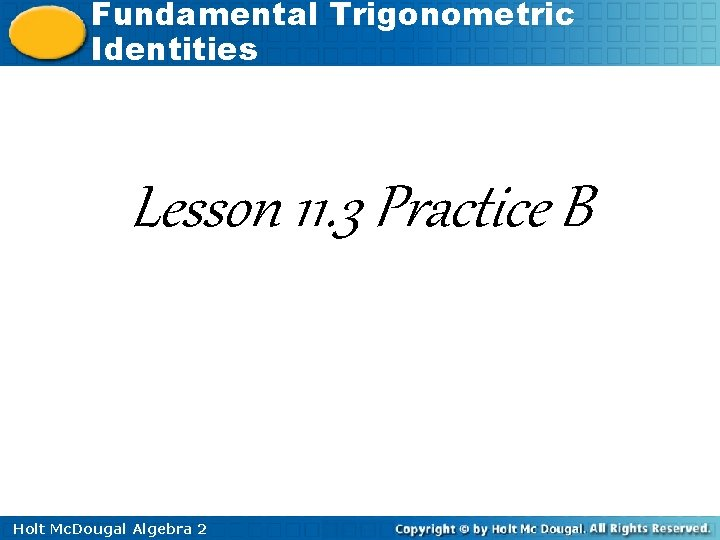Fundamental Trigonometric Identities Lesson 11. 3 Practice B Holt Mc. Dougal Algebra 2